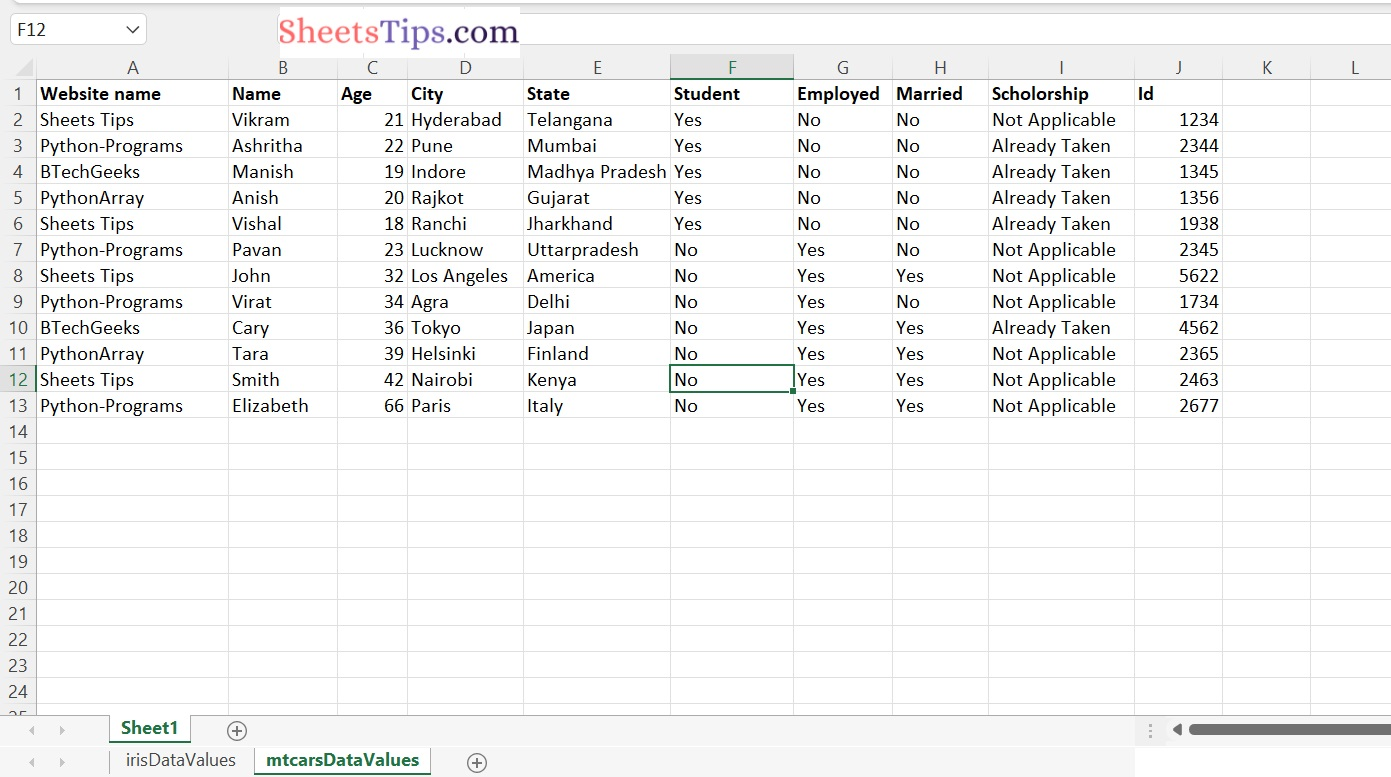# How to Convert Excel Content into DataFrame in R ?

The R Programming Language allows us to read and write data into a variety of files such as CSV, Excel, and XML.

In this tutorial, we will look at how to convert Excel data into a DataFrame in R. To read an excel file, use the read. xlsx() function of the xlsx package.

xlsx package:

The excel package is a robust Java-based language for reading, writing, and updating data from and to excel files.

Installation of xlsx package

Before we work with this package we must first install it. Type the below command in the R console:

`install.packages("xlsx")`

Output:

```Installing package into ‘C:/Users/vicky/AppData/Local/R/win-library/4.2’
(as ‘lib’ is unspecified)
also installing the dependencies ‘rJava’, ‘xlsxjars’

trying URL 'https://cloud.r-project.org/bin/windows/contrib/4.2/rJava_1.0-6.zip'
Content type 'application/zip' length 1245703 bytes (1.2 MB)

trying URL 'https://cloud.r-project.org/bin/windows/contrib/4.2/xlsxjars_0.6.1.zip'
Content type 'application/zip' length 9485708 bytes (9.0 MB)

trying URL 'https://cloud.r-project.org/bin/windows/contrib/4.2/xlsx_0.6.5.zip'
Content type 'application/zip' length 375143 bytes (366 KB)

package ‘rJava’ successfully unpacked and MD5 sums checked
package ‘xlsxjars’ successfully unpacked and MD5 sums checked
package ‘xlsx’ successfully unpacked and MD5 sums checked

The read.xlsx() function is imported from the R language’s xlsx package and used to read/import an excel file.

Syntax:

`read.xlsx("excelFilePath, sheetName = "sheet name", …)`

Parameters

excelFilePath: This is reIt is the excel file path on which we work.

sheetName: It is the name of the excel sheet to be used.

This function also has many other parameters like colNames, `rowNames`, `skipEmptyRows`, `skipEmptyCols`, `rows`, `cols`, etc., and so on to perform more modifications. These are all optional parameters.

colIndex: It is used to get only the columns that we wanted.

In this case, we set the colIndex argument to 1 to get the first column of the Excel file.

Let us use the sampleExcelFile.xlsx as an example here which is shown below:

sampleExcelFile.xlsx:## Converting Excel Content into DataFrame in R

Approach:

• Importing xlsx package using the library() function.
• Give the excel file path and store it in a variable.
• Pass the excel File , sheetName , Index of the column and store that column in a variable.
• Create a dataframe of the above 9 columns using the data.frame() function and store it in another variable.
• Print the DataFrame using the print() function.
• The Exit of the Program.

Below is the implementation:

```# Importing xlsx package using the library() function
library(xlsx)
# Give the excel file path and store it in a variable

# Pass the excel File , sheetName , Index of the column  and store that column in a variable
WebsiteName <- read.xlsx(excelfilepath, sheetName = "Sheet1", colIndex = 1)
Name <- read.xlsx(excelfilepath, sheetName = "Sheet1", colIndex = 1)
Age <- read.xlsx(excelfilepath, sheetName = "Sheet1", colIndex = 2)
City <- read.xlsx(excelfilepath, sheetName = "Sheet1", colIndex = 3)
State <- read.xlsx(excelfilepath, sheetName = "Sheet1", colIndex = 4)
Student <- read.xlsx(excelfilepath, sheetName = "Sheet1", colIndex = 5)
Employed <- read.xlsx(excelfilepath, sheetName = "Sheet1", colIndex = 6)
Married <- read.xlsx(excelfilepath, sheetName = "Sheet1", colIndex = 7)
Scholorship <- read.xlsx(excelfilepath, sheetName = "Sheet1", colIndex = 8)
Id <- read.xlsx(excelfilepath, sheetName = "Sheet1", colIndex = 9)

# Create a dataframe of the above 9 columns using the data.frame() function
# and store it in another variable
DataFrame <- data.frame(WebsiteName, Name, Age,City,State,Student,Employed,Married,Scholorship,Id)
# Print the DataFrame
print(DataFrame)```

Output:

Dataframe

```     Website.name. Website.name..1      Name Age        City          State Student Employed Married    Scholorship
1      Sheets Tips     Sheets Tips    Vikram  21   Hyderabad      Telangana     Yes       No      No Not Applicable
2  Python-Programs Python-Programs  Ashritha  22        Pune         Mumbai     Yes       No      No  Already Taken